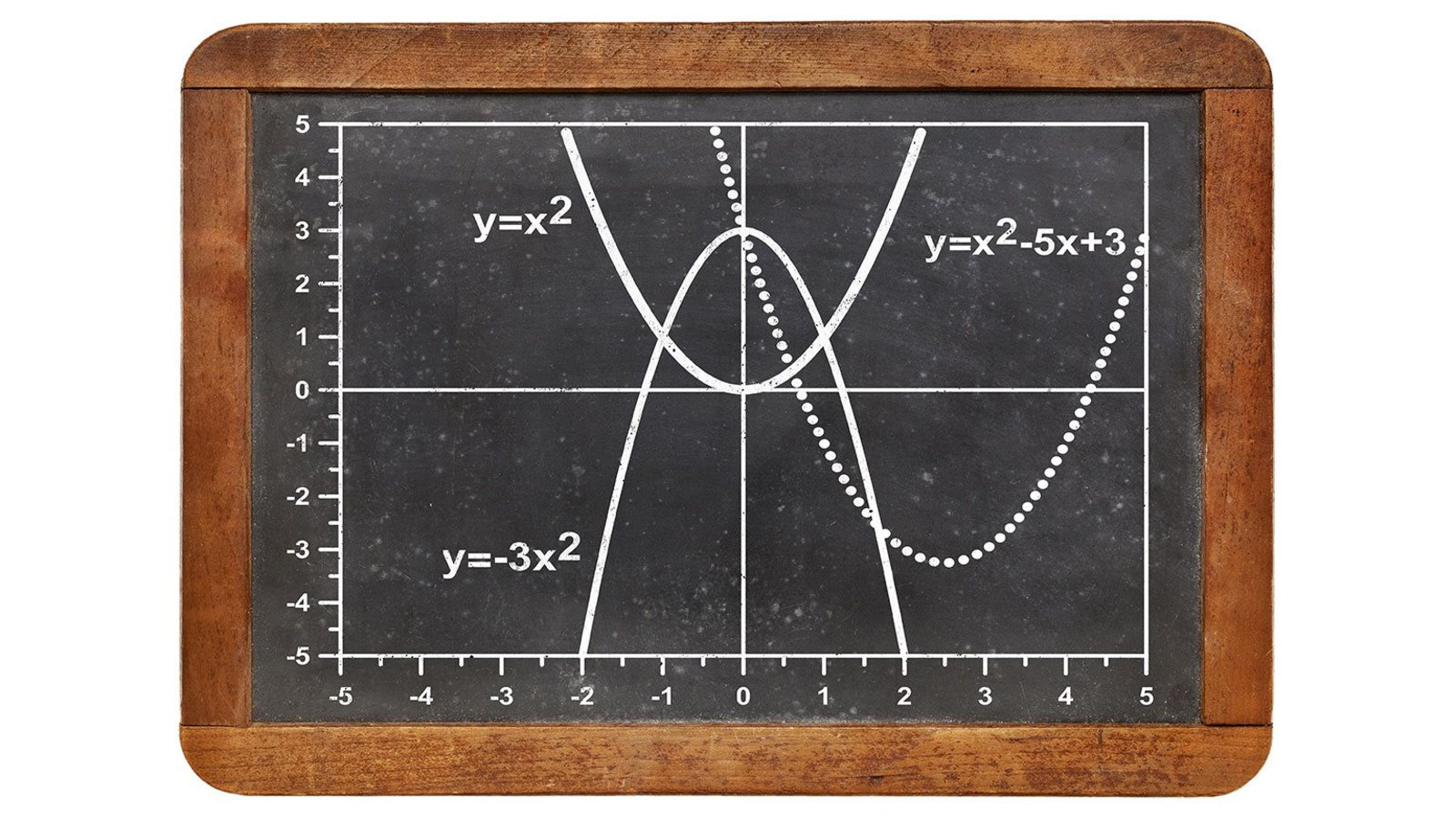The Joy of Differential Calculus Episode 18 of The Joy of Mathematics

200732 mins

Calculus is the mathematics of change, and answers questions such as: How fast is a function growing? This lecture introduces the concepts of limits and derivatives, which allow the slope of a curve to be measured at any point.

Features
Arthur T. Benjamin